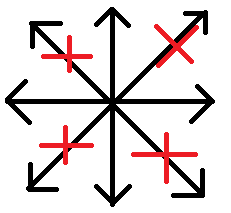# Lookat mouse in 4 directions only.

I feel like this question may have been asked before, but I could not find anything about it, so I figured I’d ask.

Now I’ve got a character which I want to look at my mouse in only four directions, so a bit like:(Sorry for my arty skills)

Now I’ve got a piece of code:

``````    private void UpdatePlayerAnimation(Vector2 Motion)
{
float MotionAngle = (float)Mathf.Atan2(Motion.y, Motion.x);

if(Motion == Vector2.zero)
{
CurrentLookSide = LookSide.Idle;
}
else if(MotionAngle >= -PiOver4 && MotionAngle <= PiOver4)
{
CurrentLookSide = LookSide.Right;
Motion = new Vector2(1, 0);
}
else if(MotionAngle >= PiOver4 && MotionAngle <= 3 * PiOver4)
{
CurrentLookSide = LookSide.Up;
Motion = new Vector2(0, 1);
}
else if(MotionAngle <= PiOver4 && MotionAngle > -3 * PiOver4)
{
CurrentLookSide = LookSide.Down;
Motion = new Vector2(0, -1);
}
else
{
CurrentLookSide = LookSide.Left;
Motion = new Vector2(-1, 0);
}
}
``````

In this the ‘Motion’ is just the velocity of my Rigidbody2D.

Now I tried moddifying the MotionAngle to match the mouse position (both the Input.mousePosition and doing a raycast), but this does not seem to work. Now my question is: am I doing something wrong (working with angles)?. My lucky guess is that the calculations are wrong for the mouse, but I can’t seem to fix it.

Anyone with some input? Thanks in advance!

P.S. I’m working in a 2D environment.

Since your object and the mouse are in two different spaces - world and screen space - you would need to translate one of these coords. E.g.

``````Camera.main.WorldToScreenPoint(transform.position);
``````

to translate the objects world coords to screen coords.

Atan2 calculates an angle from the origin to the given position. In your case you want the object to be the origin so you need to get the local mouse position relative to the object:

``````Vector2 localPosition = Input.mousePosition - Camera.main.WorldToScreenPoint(transform.position);
``````

Use the localPosition to calculate the angle. I subtract 90 deg to have 0° when the mouse is above the object:

``````float angle = (float)Mathf.Atan2(localPosition.y, localPosition.x) * Mathf.Rad2Deg - 90;
``````

Now limit the angle to the four directions 0, 90, 180, 270 - we dont want any angles between just like what Mathf.RoundToInt does if we don’t want floats. So you can use this method with a schema like 0, 1, 2, 3 what is exactly angle / 90

``````angle = Mathf.RoundToInt (angle / 90) * 90; //* 90 after rounding to get the previous schema again
``````

And finally assign the rotation

``````transform.rotation = Quaternion.Euler(new Vector3(0,0,angle));
``````